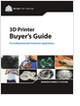Design HomeSpringsOverviewDesign ConsiderationsSpring BucklingExtension SpringsNatural FrequencyCalculatorsOverviewSpring ForceDeformed LengthSpring Rate kForce & StressRange of Rates kSpring DesignerSpring Designer EquationsCyclic Loads: ResonanceCyclic Loads: FatigueFatigue EquationsResourcesBibliography
CNC Machining Design Guide

Learn the best principles to negotiate the salary you deserve!

3D Scanners

A white paper to assist in the evaluation of 3D scanning hardware solutions.

Selecting the Right 3D Printer

Discover how to choose the right 3D printer for your needs and the key performance attributes to consider.

more free publicationsBasic CalculatorsSpring Force If you have a spring on hand and want to know its force for a given displacement, use this calculator. Spring Deflection If you have a spring and want to know the compressed length for a given applied force, use this calculator. Spring Rate k If you need to know the needed spring constant that will give you a desired spring force for a given deformed length, use this calculator.
Enriched CalculatorsForce and Stress This calculator in an extension of the basic Spring Force calculator above, providing the maximum shear stress in the spring along with the force. Range of Rate k If you only have set of design specs, consisting of a range of compressed spring lengths and a range of desired spring forces, use this calculator. It will return a range of spring constants that will meet your design specs. Spring Designer With this calculator, you can start with an idea for a spring (geometry and material data) and calculate all resulting parameters (spring rate, max load, solid height, coil pitch, etc.).Spring Resonance Use this calculator to find the first resonant frequency for a spring used in a dynamic loading environment. Spring Fatigue Use this calculator to estimate if a spring used in a dynamic loading enviroment will fail from fatigue stresses.CNC Machining Design Guide